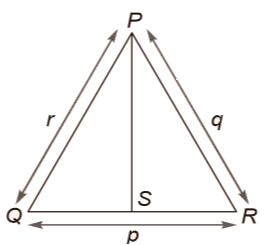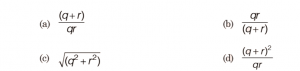Open in App
Not now

# GATE | GATE-CS-2015 (Set 2) | Question 65

• Last Updated : 28 Jun, 2021

In a triangle PQR, PS is the angle bisector of ∠ QPR and ∠ QPS = 60°. What is the length of PS?(A) a
(B) b
(C) c
(D) d

Explanation:

```Area of a Δ = 1/2 * ac sinB
= 1/2 * bc sinA
= 1/2 ab sinC

Here area (ΔPQR) = area (ΔPQS) + area (ΔPRS)

1/2 rq sin120 = 1/2 PS * r sin60 + 1/2 PS * q sin60

=> PS = rq/(r+q)```

There seems to be something wrong with question as it says ∠ QPS = 60°. The question is same on GATE official site paper.

Quiz of this Question

My Personal Notes arrow_drop_up
Related Articles